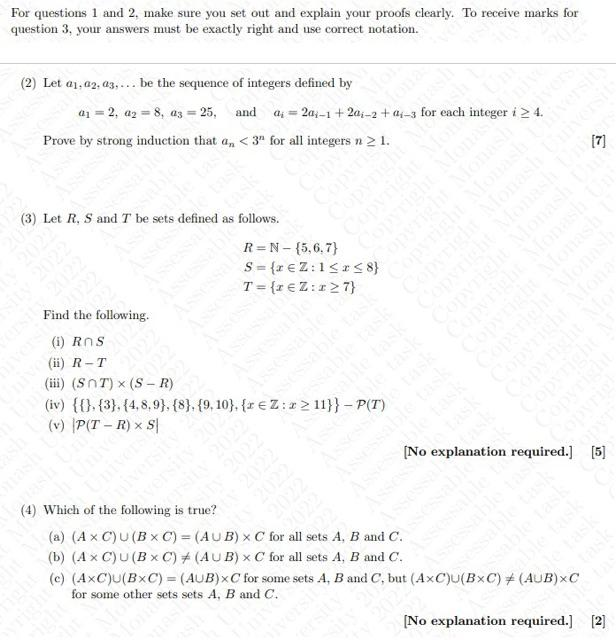Please answer questions 2, 3 and 4, thank you
Please answer questions 2, 3 and 4, thank you
Please answer questions 2, 3 and 4, thank youFor questions 1 and 2 , make sure you set out and explain your proofs clearly. To receive marks for question 3, your answers must be exactly right and use correct notation. (2) Let $$a_{1}, a_{2}, a_{3}, \ldots$$ be the sequence of integers defined by $$a_{1}=2, a_{2}=8, a_{3}=25, \quad$$ and $$\quad a_{i}=2 a_{i-1}+2 a_{i-2}+a_{i-3}$$ for each integer $$i \geq 4$$. Prove by strong induction that $$a_{n}<3^{n}$$ for all integers $$n \geq 1$$. (3) Let $$R, S$$ and $$T$$ be sets defined as follows. $\begin{array}{l} R=\mathbb{N}-\{5,6,7\} \\ S=\{x \in \mathbb{Z}: 1 \leq x \leq 8\} \\ T=\{x \in \mathbb{Z}: x \geq 7\} \end{array}$ Find the following. (i) $$R \cap S$$ (ii) $$R-T$$ (iii) $$(S \cap T) \times(S-R)$$ (iv) $$\{\{\},\{3\},\{4,8,9\},\{8\},\{9,10\},\{x \in \mathbb{Z}: x \geq 11\}\}-\mathcal{P}(T)$$ (v) $$|\mathcal{P}(T-R) \times S|$$ [No explanation required.] (4) Which of the following is true? (a) $$(A \times C) \cup(B \times C)=(A \cup B) \times C$$ for all sets $$A, B$$ and $$C$$. (b) $$(A \times C) \cup(B \times C) \neq(A \cup B) \times C$$ for all sets $$A, B$$ and $$C$$. (c) $$(A \times C) \cup(B \times C)=(A \cup B) \times C$$ for some sets $$A, B$$ and $$C$$, but $$(A \times C) \cup(B \times C) \neq(A \cup B) \times C$$ for some other sets sets $$A, B$$ and $$C$$.

We have an Answer from Expert